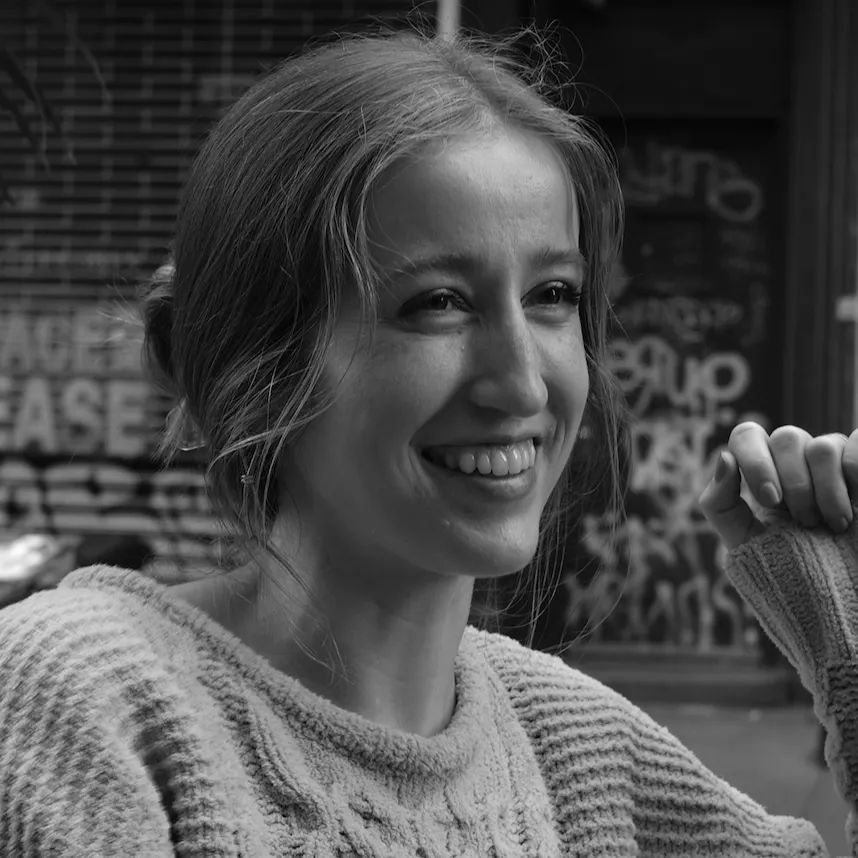Hello 2022 with Vintage Bubble Displays on the Arty Z7

As a fun start to 2022, I pulled out the HP vintage bubble displays I bought on eBay with a simple Verilog controller module on the Arty Z7.

IntermediateFull instructions provided1 hour254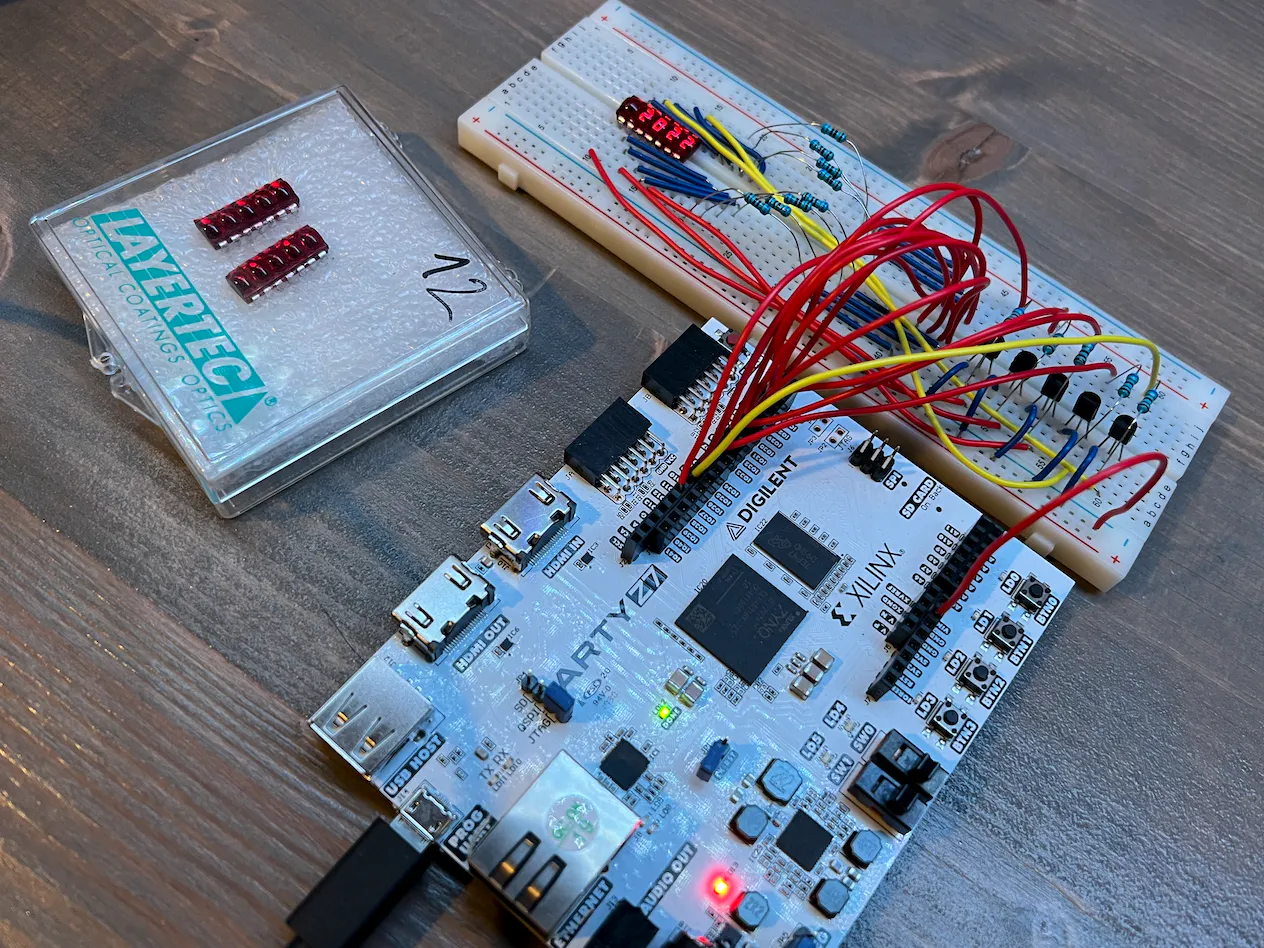Things used in this project

Hardware components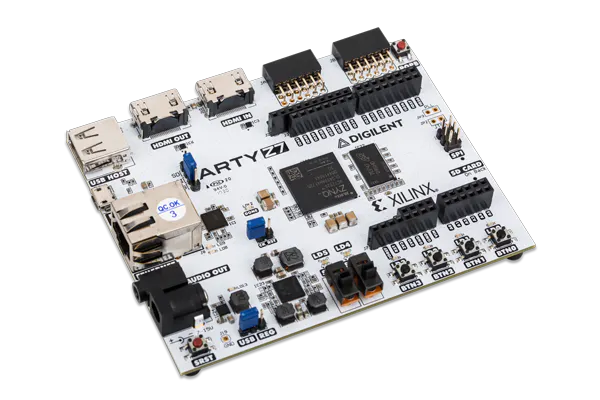Digilent Arty Z7-20
×1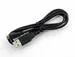USB-A to Micro-USB Cable
×1
 NPN BJT Transistor, 2N3904
×5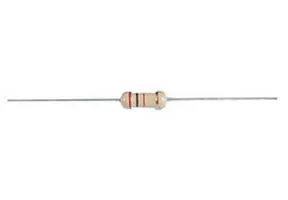Through Hole Resistor, 680 ohm
×8Through Hole Resistor, 6.8 kohm
×5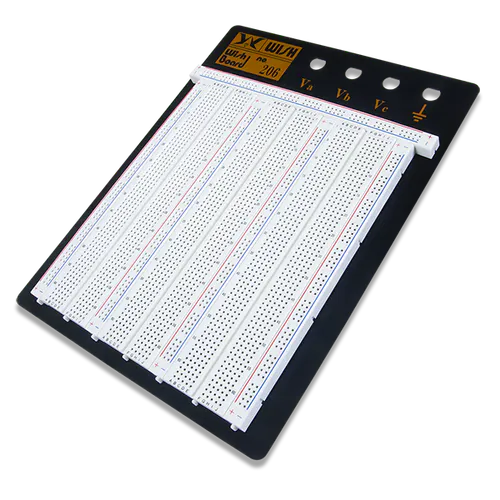×1
 HP QDSP-6061 Bubble Display (vintage)
×1

Software apps and online services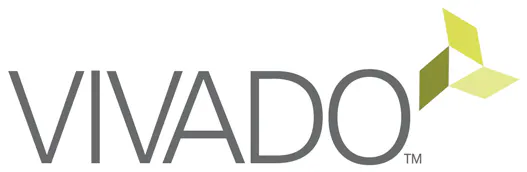Xilinx Vitis Unified Software Platform

Code

bubble_display_cntlr.v

Verilog
`timescale 1ns / 1ps
//////////////////////////////////////////////////////////////////////////////////
// Company:
// Engineer:
//
// Create Date: 12/30/2021 09:39:33 AM
// Design Name:
// Module Name: bubble_display_cntlr
// Project Name:
// Target Devices:
// Tool Versions:
// Description:
//
// This module scrolls "hello 2022" across the 5 7-segment displays of the
// HP QDSP-6061 bubble display from right to left.
//
// ---------- QDSP-6061 Pinout ----------
//
//                             a
//       d╶┐┌╴ e             ────
//      c╶┐││┌╴ f         f │    │
//     b╶┐││││┌╴ g          │  g │ b
//    a╶┐││││││┌╴ dp  ❯❯     ────
//   8'b00000000          e │    │
//                          │    │ c
//                           ────
//                             d   ■ dp
//
//    anodeReg   -> a   (pin 14)
//    anodeReg   -> b   (pin 12)
//    anodeReg   -> c   (pin  3)
//    anodeReg   -> d   (pin  6)
//    anodeReg   -> e   (pin  2)
//    anodeReg   -> f   (pin 10)
//    anodeReg   -> g   (pin  8)
//    anodeReg   -> dp  (pin  5)
//
//    N/C           -> N/C (pin 11)
//
//    cathodeReg -> c5  (pin  7)
//    cathodeReg -> c4  (pin  9)
//    cathodeReg -> c3  (pin  4)
//    cathodeReg -> c2  (pin 13)
//    cathodeReg -> c1  (pin  1)
//
//
//                       ┌╴fifth╶─────╴▮▯▯▯▯ -> c1  (pin  1)
//                       │┌╴fourth╶───╴▯▮▯▯▯ -> c2  (pin 13)
//                       ││┌╴third╶───╴▯▯▮▯▯ -> c3  (pin  4)
//                       │││┌╴second╶─╴▯▯▯▮▯ -> c4  (pin  9)
//                       ││││┌╴first╶─╴▯▯▯▯▮ -> c5  (pin  7)
// cathodeReg[4:0] <= 5'b00000
//
// Dependencies:
//
// Revision:
// Revision 0.01 - File Created
//
//////////////////////////////////////////////////////////////////////////////////

module bubble_display_cntlr(
input clk,
input reset,
output reg [7:0] anodeReg,
output reg [4:0] cathodeReg
);

parameter zero  = 8'b11111100;
parameter one   = 8'b01100000;
parameter two   = 8'b11011010;
parameter three = 8'b11110010;
parameter four  = 8'b01100110;
parameter five  = 8'b10110110;
parameter six   = 8'b10111110;
parameter seven = 8'b11100000;
parameter eight = 8'b11111110;
parameter nine  = 8'b11110110;

parameter h     = 8'b00101110;
parameter e     = 8'b10011110;
parameter l     = 8'b00011100;
parameter o     = 8'b00111010;
parameter space = 8'b00000000;

parameter cathodeC1 = 5'b00001;
parameter cathodeC2 = 5'b00010;
parameter cathodeC3 = 5'b00100;
parameter cathodeC4 = 5'b01000;
parameter cathodeC5 = 5'b10000;

parameter msec_1 = 16'd100000; // 10ns/clk -> 0.001s/0.000000010s = 100000

reg loop_incr_flag;
reg [15:0] wait_cnt;
reg [4:0]  loop_cnt;
reg [7:0]  incr_flag_cntr;
reg [7:0]  anodeReg1, anodeReg2, anodeReg3, anodeReg4, anodeReg5;

always @ (posedge clk)
begin
if (reset == 1'b0)
begin
wait_cnt <= 16'd0;
loop_incr_flag <= 1'b0;
cathodeReg <= cathodeC1;
end
else
begin
if (wait_cnt < msec_1)
begin
loop_incr_flag <= 1'b0;
wait_cnt <= wait_cnt + 1;
end
else
begin
wait_cnt <= 16'd0;

if (cathodeReg == cathodeC5)
begin
loop_incr_flag <= 1'b1;
cathodeReg <= cathodeC1;
end
else
begin
cathodeReg <= cathodeReg << 1;
end
end
end
end

always @ (posedge clk)
begin
if (reset == 1'b0)
begin
loop_cnt <= 5'd0;
incr_flag_cntr <= 8'd0;
end
else
begin
if (loop_incr_flag == 1'b1)
begin
if (incr_flag_cntr == 8'd255)
begin
if (loop_cnt == 5'd14)
begin
loop_cnt <= 5'd0;
end
else
begin
loop_cnt <= loop_cnt + 1;
end
end
else
begin
loop_cnt <= loop_cnt;
end

incr_flag_cntr <= incr_flag_cntr + 1;
end
else
begin
loop_cnt <= loop_cnt;
end
end
end

always @ (posedge clk)
begin
case (cathodeReg)
cathodeC1 : anodeReg[7:0] <= anodeReg1[7:0];
cathodeC2 : anodeReg[7:0] <= anodeReg2[7:0];
cathodeC3 : anodeReg[7:0] <= anodeReg3[7:0];
cathodeC4 : anodeReg[7:0] <= anodeReg4[7:0];
cathodeC5 : anodeReg[7:0] <= anodeReg5[7:0];
default   : anodeReg[7:0] <= anodeReg1[7:0];
endcase
end

always @ (posedge clk)
begin
case (loop_cnt)
0 :
begin
anodeReg1[7:0] <= space;
anodeReg2[7:0] <= space;
anodeReg3[7:0] <= space;
anodeReg4[7:0] <= space;
anodeReg5[7:0] <= space;
end
1 :
begin
anodeReg1[7:0] <= space;
anodeReg2[7:0] <= space;
anodeReg3[7:0] <= space;
anodeReg4[7:0] <= space;
anodeReg5[7:0] <= h;
end
2 :
begin
anodeReg1[7:0] <= space;
anodeReg2[7:0] <= space;
anodeReg3[7:0] <= space;
anodeReg4[7:0] <= h;
anodeReg5[7:0] <= e;
end
3 :
begin
anodeReg1[7:0] <= space;
anodeReg2[7:0] <= space;
anodeReg3[7:0] <= h;
anodeReg4[7:0] <= e;
anodeReg5[7:0] <= l;
end
4 :
begin
anodeReg1[7:0] <= space;
anodeReg2[7:0] <= h;
anodeReg3[7:0] <= e;
anodeReg4[7:0] <= l;
anodeReg5[7:0] <= l;
end
5 :
begin
anodeReg1[7:0] <= h;
anodeReg2[7:0] <= e;
anodeReg3[7:0] <= l;
anodeReg4[7:0] <= l;
anodeReg5[7:0] <= o;
end
6 :
begin
anodeReg1[7:0] <= e;
anodeReg2[7:0] <= l;
anodeReg3[7:0] <= l;
anodeReg4[7:0] <= o;
anodeReg5[7:0] <= space;
end
7 :
begin
anodeReg1[7:0] <= l;
anodeReg2[7:0] <= l;
anodeReg3[7:0] <= o;
anodeReg4[7:0] <= space;
anodeReg5[7:0] <= two;//k;
end
8 :
begin
anodeReg1[7:0] <= l;
anodeReg2[7:0] <= o;
anodeReg3[7:0] <= space;
anodeReg4[7:0] <= two;
anodeReg5[7:0] <= zero;
end
9 :
begin
anodeReg1[7:0] <= o;
anodeReg2[7:0] <= space;
anodeReg3[7:0] <= two;
anodeReg4[7:0] <= zero;
anodeReg5[7:0] <= two;
end
10 :
begin
anodeReg1[7:0] <= space;
anodeReg2[7:0] <= two;
anodeReg3[7:0] <= zero;
anodeReg4[7:0] <= two;
anodeReg5[7:0] <= two;
end
11 :
begin
anodeReg1[7:0] <= two;
anodeReg2[7:0] <= zero;
anodeReg3[7:0] <= two;
anodeReg4[7:0] <= two;
anodeReg5[7:0] <= space;
end
12 :
begin
anodeReg1[7:0] <= zero;
anodeReg2[7:0] <= two;
anodeReg3[7:0] <= two;
anodeReg4[7:0] <= space;
anodeReg5[7:0] <= space;
end
13 :
begin
anodeReg1[7:0] <= two;
anodeReg2[7:0] <= two;
anodeReg3[7:0] <= space;
anodeReg4[7:0] <= space;
anodeReg5[7:0] <= space;
end
14 :
begin
anodeReg1[7:0] <= two;
anodeReg2[7:0] <= space;
anodeReg3[7:0] <= space;
anodeReg4[7:0] <= space;
anodeReg5[7:0] <= space;
end

default :
begin
anodeReg1[7:0] <= h;
anodeReg2[7:0] <= e;
anodeReg3[7:0] <= l;
anodeReg4[7:0] <= l;
anodeReg5[7:0] <= o;
end
endcase
end

endmodule

bubble_display_pinout.xdc

XML
## ChipKit Outer Digital Header - J4
set_property -dict { PACKAGE_PIN T14   IOSTANDARD LVCMOS33 } [get_ports { bubble_display_cathodeReg }]; #IO_L5P_T0_34            Sch=CK_IO0   QDSP-6061 pin 1
set_property -dict { PACKAGE_PIN U12   IOSTANDARD LVCMOS33 } [get_ports { bubble_display_anodeReg   }]; #IO_L2N_T0_34            Sch=CK_IO1   QDSP-6061 pin 2
set_property -dict { PACKAGE_PIN U13   IOSTANDARD LVCMOS33 } [get_ports { bubble_display_anodeReg   }]; #IO_L3P_T0_DQS_PUDC_B_34 Sch=CK_IO2   QDSP-6061 pin 3
set_property -dict { PACKAGE_PIN V13   IOSTANDARD LVCMOS33 } [get_ports { bubble_display_cathodeReg }]; #IO_L3N_T0_DQS_34        Sch=CK_IO3   QDSP-6061 pin 4
set_property -dict { PACKAGE_PIN V15   IOSTANDARD LVCMOS33 } [get_ports { bubble_display_anodeReg   }]; #IO_L10P_T1_34           Sch=CK_IO4   QDSP-6061 pin 5
set_property -dict { PACKAGE_PIN T15   IOSTANDARD LVCMOS33 } [get_ports { bubble_display_anodeReg   }]; #IO_L5N_T0_34            Sch=CK_IO5   QDSP-6061 pin 6
set_property -dict { PACKAGE_PIN R16   IOSTANDARD LVCMOS33 } [get_ports { bubble_display_cathodeReg }]; #IO_L19P_T3_34           Sch=CK_IO6   QDSP-6061 pin 7
set_property -dict { PACKAGE_PIN U17   IOSTANDARD LVCMOS33 } [get_ports { bubble_display_anodeReg   }]; #IO_L9N_T1_DQS_34        Sch=CK_IO7   QDSP-6061 pin 8
set_property -dict { PACKAGE_PIN V17   IOSTANDARD LVCMOS33 } [get_ports { bubble_display_cathodeReg }]; #IO_L21P_T3_DQS_34       Sch=CK_IO8   QDSP-6061 pin 9
set_property -dict { PACKAGE_PIN V18   IOSTANDARD LVCMOS33 } [get_ports { bubble_display_anodeReg   }]; #IO_L21N_T3_DQS_34       Sch=CK_IO9   QDSP-6061 pin 10
set_property -dict { PACKAGE_PIN T16   IOSTANDARD LVCMOS33 } [get_ports { bubble_display_anodeReg   }]; #IO_L9P_T1_DQS_34        Sch=CK_IO10  QDSP-6061 pin 12
set_property -dict { PACKAGE_PIN R17   IOSTANDARD LVCMOS33 } [get_ports { bubble_display_cathodeReg }]; #IO_L19N_T3_VREF_34      Sch=CK_IO11  QDSP-6061 pin 13
set_property -dict { PACKAGE_PIN P18   IOSTANDARD LVCMOS33 } [get_ports { bubble_display_anodeReg   }]; #IO_L23N_T3_34           Sch=CK_IO12  QDSP-6061 pin 14

Credits

Whitney Knitter

62 projects • 787 followers
Working as a full-time SDR/FPGA engineer, but making time for the fun projects at home.